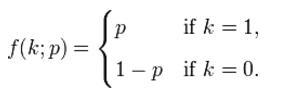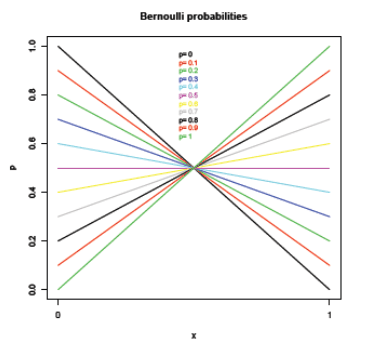# Properties of Bernoulli Distribution

Definition

The Bernoulli distribution is a discrete probability distribution which consists of Bernoulli trials.

Each Bernoulli trial has the following characteristics:

• There are only two outcomes a 1 or 0, i.e., success or failure each time.
• If the probability of success is p then the probability of failure is  1-p and this remains the same across each successive trial.
• The probabilites are not affected by the outcomes of other trials which means the trials are independent.

The probability mass function commonly abbreviated as by PMF is given by:The graph of the Bernoulli probabilities is as depicted below:An example that best illustrates the Bernoulli distribution is a single toss of a coin. Every successive toss is independent of the previous tosses when it comes to determining the outcome.

In case there are more than one trial or in case of many trials the Bernoulli distribution extends to the Binomial distribution. This is then called a Binomial experiment and gives rise to a binomial random variable.

The key characteristics of a binomial experiment are as follows:

• A number of Bernoulli trials are to be performed under one experiment and these are pre-determined.
• Each trial has an experiment whereby there are two labelled outcomes success or failure,
• The probability of success in each trial is the same.
• The trials are independent of each other which mean that one trial’s outcome is not affected by the outcome of any other trial.

History and relevance

• Swiss scientist Jacob Bernoulli is accredited with the invention of this distribution and he also came up with the idea of the Binomial distribution.
• It is used in situations where a random variable is associated with two outcomes.

Properties

The expected value of the random variable is given by E(x) = p and can be derived as follows

E(x) = 0*(1-p) + 1 * p = p

The variance of the Bernoulli variable is given by p*(1-p) and is given as follows:

Var(X) = p - p2 = p*(1-p)

Applications

• There are real life situations which involve noting if a specific event occurs or not which is recorded as a success or a failure. The Bernoulli distribution finds application in this situation.
• Some examples that best explain such scenarios are the probability of getting a head in a single coin flip, probability of having a boy child or the probability of getting a hike in the salary package.